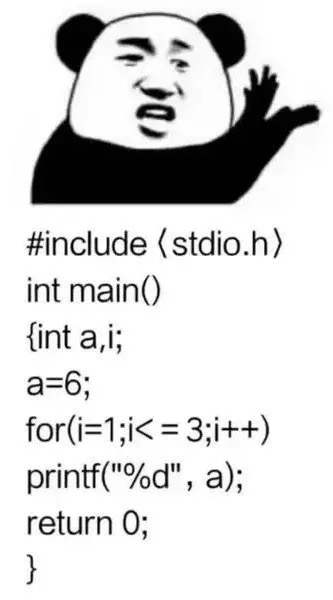# 置顶反复横跳的瞄准线！ 从向量计算说起！基于射线检测的实现！精选热门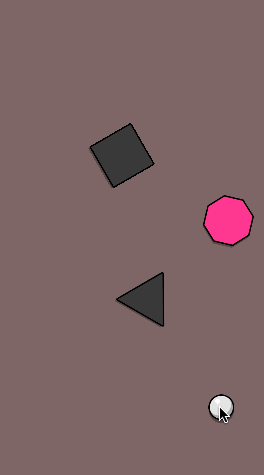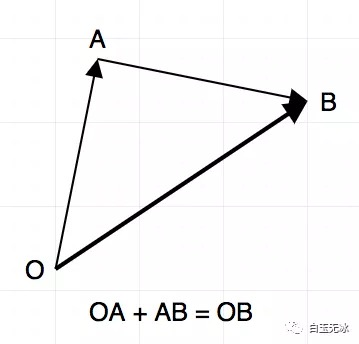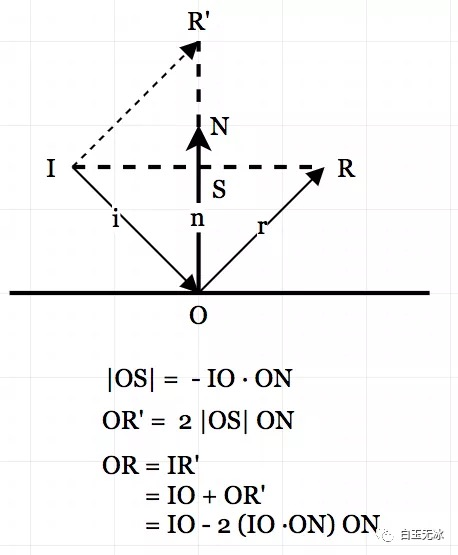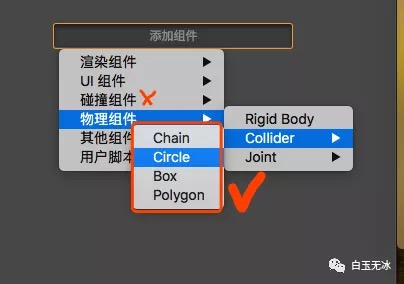```cc.director.getPhysicsManager().enabled = true
```

```/**
* @description 计算射线
* @param startLocation 起始位置 世界坐标系
* @param vector_dir 单位方向向量
*/privatedrawRayCast(startLocation: cc.Vec2, vector_dir: cc.Vec2){
// 剩余长度const left_length = AIM_LINE_MAX_LENGTH - this._cur_length;
if (left_length <= ) return;
// 射线测试const results = cc.director.getPhysicsManager().rayCast(startLocation, endLocation, cc.RayCastType.Closest);
if (results.length > ) {
const result = results[];
// 指定射线与穿过的碰撞体在哪一点相交。const point = result.point;
// 画入射线段this.drawAimLine(startLocation, point);
// 计算长度const line_length = point.sub(startLocation).mag();
// 计算已画长度this._cur_length += line_length;
// 指定碰撞体在相交点的表面的法线单位向量。const vector_n = result.normal;
// 入射单位向量const vector_i = vector_dir;
// 反射单位向量const vector_r = vector_i.sub(vector_n.mul( * vector_i.dot(vector_n)));
// 接着计算下一段this.drawRayCast(point, vector_r);
} else {
// 画剩余线段this.drawAimLine(startLocation, endLocation);
}
}
```

```/**
* @description 画瞄准线
* @param startLocation 起始位置 世界坐标系
* @param endLocation 结束位置 世界坐标系
*/privatedrawAimLine(startLocation: cc.Vec2, endLocation: cc.Vec2){
// 转换坐标
const graphic_startLocation = this.graphic_line.node.convertToNodeSpaceAR(startLocation;
this.graphic_line.moveTo(graphic_startLocation, graphic_startLocation;
// 画小圆圆// 间隔
const delta = 20;
// 方向
const vector_dir = endLocation.sub(startLocation);
// 数量
const total_count = Mathround(vector_dir.mag() / delta);
// 每次间隔向量
vector_dir.normalizeSelf().mulSelf(delta;
for (let index = ; index < total_count; index++) {